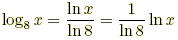# change of base formula

Change of Base Formula

A formula that allows you to rewrite a logarithm in terms of logs written with another base. This is especially helpful when using a calculator to evaluate a log to any base other than 10 or e.

 Assume that x, a, and b are all positive. Also assume that a ≠ 1, b ≠ 1.Change of base formula: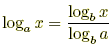Example 1: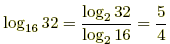Example 2: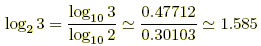(note that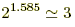) Example 3: